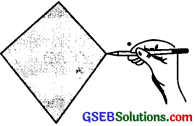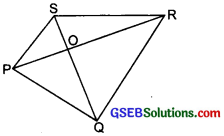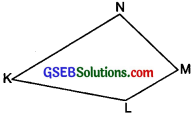# GSEB Solutions Class 6 Maths Chapter 4 Basic Geometrical Ideas Ex 4.5

Gujarat Board GSEB Textbook Solutions Class 6 Maths Chapter 4 Basic Geometrical Ideas Ex 4.5 Textbook Questions and Answers.

## Gujarat Board Textbook Solutions Class 6 Maths Chapter 4 Basic Geometrical Ideas Ex 4.5

Question 1.
Draw a rough sketch of a quadrilateral PQRS. Draw its diagonals. Name them. Is the meeting point of the diagonals in the interior or exterior of the quadrilateral?Solution:
(i) The figure is a quadrilateral PQRS.
(ii) Its diagonals are : $$\overline{\mathrm{OS}}$$ and $$\overline{\mathrm{PR}}$$.
(iii) The meeting point of these diagonals O is in the interior of the quadrilateral.Question 2.
Draw a rough sketch of a quadrilateral KLMN State:
(a) two pairs of opposite sides,
(b) two pairs of opposite angles,
(c) two pairs of adjacent sides,
(d) two pairs of adjacent angles.
Solution:
(a) The figure KLMN is a quadrilateral. Its two pairs of opposite sides are:$$\overline{\mathrm{KL}}$$, $$\overline{\mathrm{MN}}$$ and $$\overline{\mathrm{LM}}$$, $$\overline{\mathrm{NK}}$$

(b) Its two pairs of opposite angles are:
$$\angle K$$, $$\angle M$$ and $$\angle L$$, $$\angle N$$

(e) Its two pairs of adjacent sides are:
$$\overline{\mathrm{KL}}$$, $$\overline{\mathrm{LM}}$$ and $$\overline{\mathrm{KN}}$$, $$\overline{\mathrm{NM}}$$

(d) Its two pairs of adjacent angles are:
$$\angle K$$, $$\angle L$$ and $$\angle L$$, $$\angle M$$Question 3.
Investigate: Use strips and fasteners to make a triangle and a quadrilateral. Try to push in ward at any one vertex of the triangle. Do the same for the quadrilateral. Is the triangle distorted? Is the quadrilateral distorted? is the triangle rigid? Why is it that structures like electronic towers make use of triangular shapes and not quadrilaterals?
Solution:
Do it yourself.